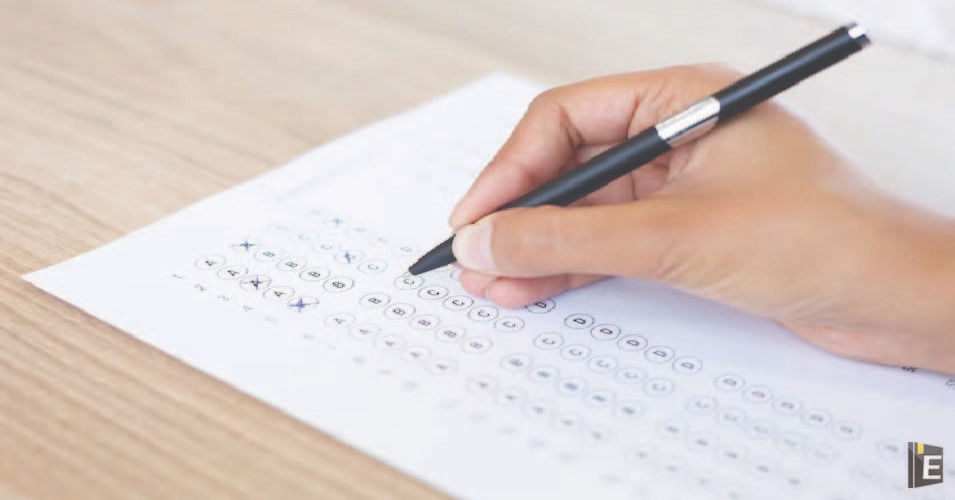• Save

# Basic Mathematics Questions and AnswersWelcome to our Basic Mathematics Questions and Answers Blog, your go-to resource for understanding fundamental mathematical concepts and solving common math problems. Whether you're a student seeking clarity or someone looking to brush up on essential math skills, this blog is designed to provide concise explanations and practical solutions. Our team of math enthusiasts and educators curates a wide range of topics, covering arithmetic, algebra, geometry, trigonometry, and more. Each blog post focuses on a specific concept or problem type, breaking it down into easy-to-understand steps. We aim to make math accessible to all, using clear language and visual aids when necessary.

In our blog Basic Mathematics Questions and Answers, you'll find a variety of questions that cover both theory and application. From solving equations and calculating percentages to understanding geometric shapes and applying mathematical principles to real-life situations, we strive to address the core areas of basic math. Our goal is to help you build a strong foundation in mathematics and develop problem-solving skills that can be applied in various academic and everyday scenarios.

Master the Quiz: Embark on a Journey of Learning with our Dynamic General Knowledge Mock Test and Current Affairs Mock Test!"

Also, Read Latest Current Affairs Questions 2023: Current Affairs Today

## Basic Mathematics Questions and Answers

Q :

what is the value of the expression
cos 2A cos2B+sin2(A-B)-sin2(A+B)?

(A) sin(2A-2B)

(B) cos(2A+2B)

(C) cos(2A-2B)

(D) sin(2A+2B)

Q :

if a=26 and b=22, then the value of   $${{a^3-b^3}\over {a^2-b^2}}-{3ab\over a+b}$$ is

(A) $$11\over 13$$

(B) $$1\over 3$$

(C) $$5\over 3$$

(D) $$13\over 11$$

Q :

In △ABC, D and E are points on sides AB and AC, such that DE||BC. If AD=x+3, DB=2x-3, AE=x+1 and EC=2x-2, then the value of x is

(A) $$1\over 5$$

(B) $$4\over 5$$

(C) $$3\over 5$$

(D) $$1\over 2$$

Q :

The minute hand of a clock is 20 cm long. Find the area on the face of the clock swept by the minute hand between 8 a.m. and 8:45 a.m.

(A) $${6600\over 18}cm^2$$

(B) $${6600\over 7}cm^2$$

(C) $${6600\over 14}cm^2$$

(D) $${6600\over 9}cm^2$$

Q :

If sin2θ - 3sinθ + 2=0, the find the value of θ(00≤ θ≤900)

(A)

450

(B)

00

(C)

600

(D)

900

Q :

What is the rate of interest per annum for simple interest at which Rs.880 amounts to Rs.913 in $$1{1\over 2}$$years?

(A) $$2{2\over 3}\%$$

(B) $$2{1\over 4}\%$$

(C) $$2{1\over 2}\%$$

(D) $$2{1\over 3}\%$$

Q :

if $$a+{1\over a}=5$$, then what is the value of $$a^3+{1\over a^3}$$

(A) 110

(B) 15

(C) 105

(D) -10

Q :

Raju, Shobha and Mohan can do a work in 15 days, 20 days and 25 days respectively. In how many days, will the work be finished, if they do it on alternate days?

(A) $$15{9\over 10}$$ days

(B) $$18{7\over 10}$$ days

(C) $$21{7\over 10}$$ days

(D) $$18{9\over 10}$$ days

Q :

What is the value of a2+b2+c2-2ab-2bc+2ca?

(A) (2a + b + c)2

(B)

(a − b + c)2

(C)

(a − b − 2c)2

(D)

(a + 2b − c)2

Q :

△ABC is right-angle triangle at B and tan A = $$3\over 4$$, then sin A + sin B + sin C will be equal to:

(A) $$2{4\over 5}$$

(B) $$2{2\over 5}$$

(C) $$3{1\over 5}$$

(D) $$1{1\over 5}$$